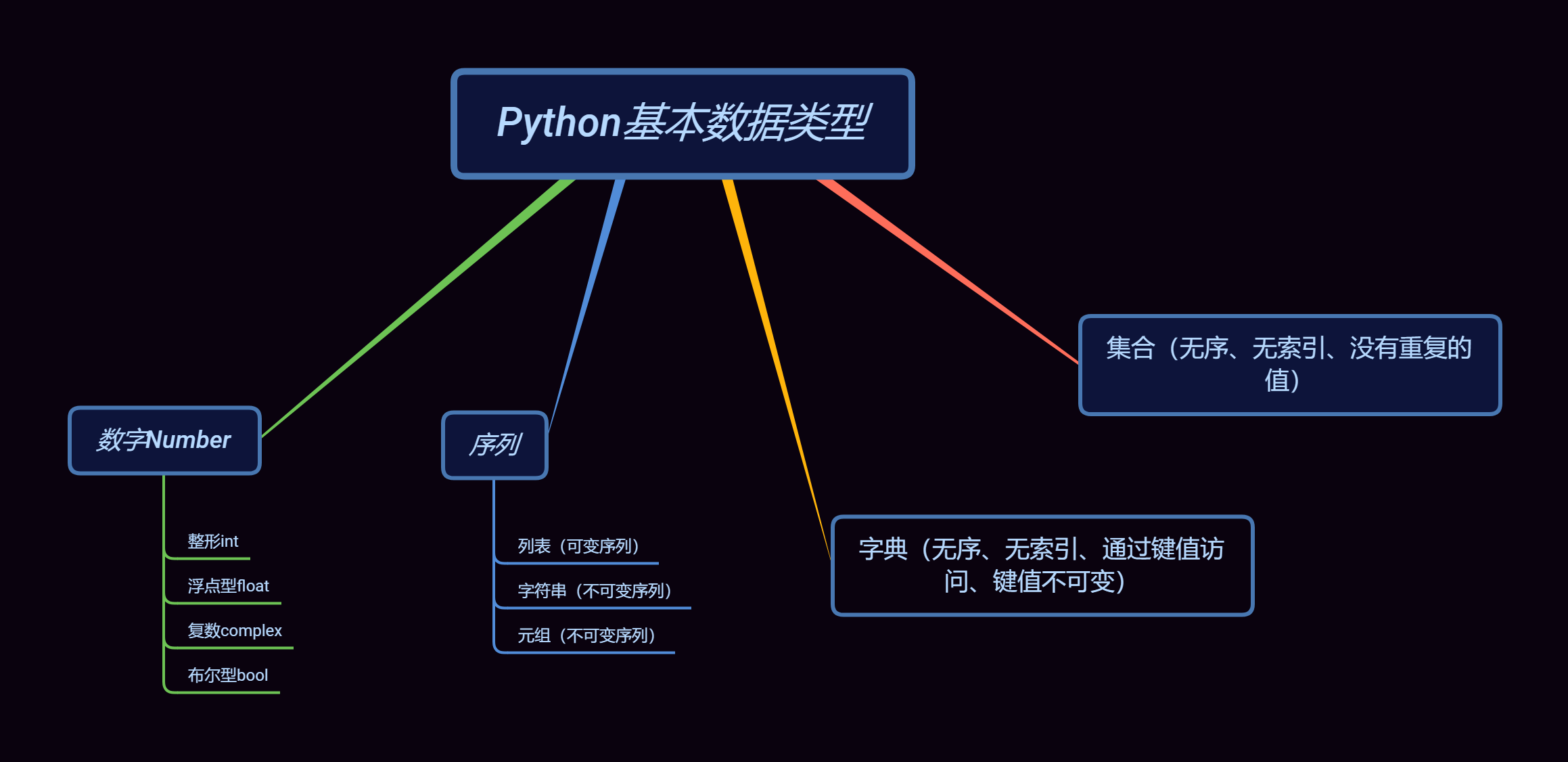# Python基本数据类型

### Python基本数据类型type()：查看数据的类型
isinstance（值，类型名），判断值是否为填上的数据类型

# 数字Number

Python3 支持
1.整型int
2.浮点数float
3.复数complex
4.布尔型bool

• 整形int

Python3 整型是没有限制大小的，可以当作 Long 类型使用，所以 Python3 没有 Python2 的 Long 类型

• 浮点型float

• 复数complex

复数语法：complex（[ real [，imag ] ]）

real 可以为int、float或字符串类型；而 imag 只能为int、float类型。

• 布尔类型bool

None、数字类型中的 0、空字符串“”、空元组()、空列表[]、空字典{}、自定义类型，实现了nonzero()或len()方法并且方法返回 0 或 False，则都也被当作False

# 序列

### 列表List

列表中各元素间是有序的，属于序列类型。列表可以进行元素的 添加，删除，查找，替换 等操作。列表长度没有限制，不需要定义长度，列表中的元素类型可以不同，可以是 字母，数字，集合，列表，元组，字符串等。list类似C语言中的数组，用于顺序存储结构

list()生成一个空列表，或是转化为列表类型
extend()在列表末尾分别添加元素
append()在列表末尾添加一整个元素
clear()删除列表中的所有元素
copy()生成一个新列表，复制所有元素。
count()统计列表中元素出现的次数
index()返回第一次出现元素的位置
insert()在列表下标X的位置，添加元素
pop()将列表第X项元素取出，并删除该元素
remove()将列表中出现的第一个元素删除。
reverse()将列表ls中的元素反转。
sort()将列表中的同类元素按从小到大排序
1. list（）
#第一种，创建空列表
a =  list（）
#第二种 直接创建列表赋值
a = [值，值，值]

1. extend()
a = ['我爱你', '荆鑫']
b = [1,3,1,4, 5,2,0]
a.extend(b) #返回值为空，将列表 b 的元素添加到列表 a 末尾。
print(a)
print(b) #列表 b 元素不变
#执行结果
#['我爱你', '荆鑫', 1, 3, 1, 4, 5, 2, 0]
#[1, 3, 1, 4, 5, 2, 0]

1. append()
a = ["我爱你", "荆鑫"]
b = [1,3,1,4, 5,2,0]
a.append(b)
print(a)
#执行结果
#['我爱你', '荆鑫', [1, 3, 1, 4, 5, 2, 0]]


extend（）是把所有元素都添加到末尾。
append（）是把括号里包含的所有元素当成一整体添加进末尾变成元素。

1. clear()
a = ["我爱你", "荆鑫"]
a.clear()
print(a)
#执行结果返回空列表
#[]

1. copy()
a = ["我爱你", "荆鑫"]
b = 
c = a.copy()#c 复制 a 中的所有元素
a.clear()#删除 a 中所有元素
a = b#这不是复制，而是给列表 b 新关联一个引用，即增加一个别名，b 和 a 指向同一个内存地址
print(a + c)
print(id(a), id(b))
print(id(c))
#执行结果
#[520, '我爱你', '荆鑫']
#1814915539592 1814915539592
#1814916764680


1. 修改原列表内嵌套列表的元素，还是修改复制列表的内嵌套列表元素。他们的内嵌套列表元素都将改变。
2. 修改原列表和复制列表的其它非嵌套元素，都不改变对方相应的元素。
a = [ '我爱你', '荆鑫', [1314, 520]]
b = a.copy()#b复制 a 中的所有元素
a = "我喜欢你"#对原列表 a 中的第一个元素进行修改
a = 'love'#对原列表 中第3个元素的第1个元素进行修改
print(a)#复制到列表 b 的第1个元素不变，但第3个元素的第1个元素发生改变。
print(b)
#执行结果
#['我喜欢你', '荆鑫', ['love', 520]]
#['我爱你', '荆鑫', ['love', 520]]

1. count()
a = ['荆鑫', 0,3,0,6, '我', 0,9,2,9]
print(a.count(0)) #统计列表中 0 出现的次数
print(a.count(9)) #统计列表中 9 出现的次数
#执行结果
#3
#2

1. index()
ls = [1,2,3,"a",3,5,"a",5,[1,7,"b"]]
print(ls.index("a"))
#返回列表ls中"a"第一次出现的位置。
print(ls.index("a",4))
#索引的起始位置为下标为4的元素，索引范围为（ 3, 5, 'a', 5, [1, 7, 'b']）
print(ls.index("a",4,8))
#索引的起始位置为下标为 4 的元素,结束位置为下标为 7 的元素。索引范围( 3, 5, 'a', 5)
print(ls.index("b"))
#返回列表ls内嵌套列表[1,7,"b"]中"b"第一次出现的位置
#执行结果
#3
#6
#6
#2

1. insert()
ls = [1,3,1,["荆","鑫"]]
ls.insert(3,"520")#在列表ls下标为3的位置插入元素"520"
ls.insert(3,4) #在列表ls下标为3的位置插入元素 4
ls.insert(0,"爱你") #在列表ls的内嵌套列表["a",5,8]，下标为0的位置插入字符串"acv"
print(ls)
#执行结果
#[1, 3, 1, 4, '520', ['爱你', '荆', '鑫']]

1. pop()
ls = [1,3,1,4,"荆","鑫"]
print(ls.pop(0)) #取出下标为0的元素，并从列表删除。
print(ls.pop(2))#取出下标为3的元素，并从列表删除。
print(ls.pop()) #默认取出最后一个元素，并删除。
print(ls)
#执行结果
#1
#4
#鑫
#[3, 1, '荆']

1. remove()
ls1 = [1,2,1,2,"a",3,"a"]
ls2 = [1,2,1,2,"a",3,"a"]
ls1.remove(1) #删除ls1中第一次出现的元素 1
ls2.remove("a") ##删除ls2中第一次出现的元素 "a"
print(ls1)
print(ls2)
#执行结果
[2, 1, 2, 'a', 3, 'a']
[1, 2, 1, 2, 3, 'a']

1. reverse()
ls1 = [1,2,3,4,5,6,7,8,9]
ls1.reverse() #将列表ls1反转输出
print(ls1)
#执行结果
#[9, 8, 7, 6, 5, 4, 3, 2, 1]

1. sort()
ls1 = [2,5,8,9,4,1,2,6,2,1,3]
ls1.sort()#默认将列表ls2按从小到大的序数排列。
print(ls1)
#执行结果
#[1, 1, 2, 2, 2, 3, 4, 5, 6, 8, 9]


### 字符串Str

strip()去掉字符串头尾空白符
startswith() \ endswith()判断字符串是否以指定字符开头 \ 结尾 (返回布尔型)
find() \ rfind()查找字符串中指定值首次 \ 末次的位置，返回下标
replace()返回 new 替换 old 的新字符串
len()返回字符串的长度
count()统计字符串里某个字符出现的次数
upper() \ lower()返回字符串的所有字母大写 \ 小写的形式
center()返回固定字符串长度，用自选符号填充两边
format()返回一个格式化的字符串,控制字符串的显示效果。
1. strip()lsprip() 和 rstrip()

strip()

1. 括号为空：默认去掉头尾空白符（\n、\r、\t、‘空格’）
2. 括号有值：把其值拆成一个个字符，一一对应头尾字符，知道遇上非匹配为止
a = " \n 123456789 \r"
b = " 1234567893 "
print(a.strip())#去掉头尾的制表符空白符等
print(b.strip("123 "))#出现第一个括号里的字符不匹配就停止
#执行结果
#123456789
#456789


lsprip() 和 rstrip()

lsprip()：去掉左边字符
rstrip()：去掉右边字符

a = " 1234567893 "
print(a.lstrip("123 "))#去掉左边字符"123 "
print(a.rstrip("123 "))#去掉右边字符"123 "
#执行结果
#4567893
# 123456789

1. startswith() \ endswith()
a = "1234567893 "
print(a.startswith("123"))#判断左边开头字符是否为"123 "
print(a.endswith("123"))##判断右边结尾字符是否为"123 "
#执行结果
#True
#False

1. find() \ rfind()index()

a = "1224557993 "
print(a.index('0'))#查询不到报错
print(a.index('22'))#从头查询字符"22 "，返回第一个的下标
print(a.find('0'))#查询不到，返回-1
print(a.find("22"))#从头查询字符"22 "，返回第一个的下标
print(a.rfind("9"))#从末尾查询字符"9 "，返回末尾到开头第一个的下标
print(a.rfind("99"))#从末尾查询字符"9 "，返回开头到末尾第一个的下标
#执行结果
#显示报错
#1
#-1
#1
#8
#7

1. replace()

replace(old，new，count)

a = "520荆鑫"
print(a.replace("520","1314"))
#执行结果
#1314荆鑫

1. len()
a = "520荆鑫"
print(len(a))
#执行结果
#5

1. count()
a = "1314520荆鑫 "
print(a.count("1"))
#执行结果
#2

1. upper() 和 lower()
a = "abcdefGHJKL"
print(a.upper())
print(a.lower())
#执行结果
#ABCDEFGHJKL
#abcdefghjkl

1. center()
a = "1314荆鑫"
print(a.center(10,"-"))#用 - 来填充两边多余的长度
print(a.center(5,"-"))#字符串长度大于5，不起效果
#执行结果
#--1314荆鑫--
#1314荆鑫

1. center()
a = "1314荆鑫"
print(a.center(10,"-"))#用 - 来填充两边多余的长度
print(a.center(5,"-"))#字符串长度大于5，不起效果
#执行结果
#--1314荆鑫--
#1314荆鑫

1. format()
print("\n1.默认方法")
a = "1314{},荆鑫{}"
print(a.format("520","我爱你"))#有多个槽{}，照槽{}出现顺序一一对应format()函数中的不同参数
print("\n2.序号方法")
b = "1314{0},荆鑫{0}"
print(b.format("我爱你"))#全部序号一致，代表一个参数对应全部
b = "1314{0},荆鑫{1}"
print(b.format("520","我爱你"))#序号可以切换不一样的值
b = "1314{1},荆鑫{0}"
print(b.format("520","我爱你"))
print("\n3.参数方法")
b = "{who}爱你，{jx}，13{time}"
print(b.format(who="我",jx="荆鑫",time="14"))  #通过对槽{}命名，命名赋值
#执行结果
#
#1.默认方法
#1314520,荆鑫我爱你
#
#2.序号方法
#1314我爱你,荆鑫我爱你
#1314520,荆鑫我爱你
#1314我爱你,荆鑫520
#
#3.参数方法
#我爱你，荆鑫，1314


### 元组Tuple

1. a = ()
2. b = tuple()

a = (1)
print(type(a))
a = (1,)
print(type(a))#注意，一个参数的元组有逗号
b = (1,2)
print(type(b))
#执行结果
#<class 'int'>
#<class 'tuple'>
#<class 'tuple'>


len()返回元组数量
max()返回元组最大值
min()返回元组最小值
a = (1,2,3,4,5,6,7,8)
print(len(a))
print(max(a))
print(min(a))
#执行结果
#8
#8
#1


# 字典Dict

字典是无序的，只能通过键访问成员，而不能通过序号位置。
字典的键是不可变的，如字符串、数字和元组数据类型，值可以为任何数据类型。

1. {}
2. dIct{}

1. 删除键值
del 字典名 [ “键1” ]
2. 清除字典数据
变量名 . clear（）
3. 删除整个字典
del 变量名

a = {“键1”：值1，“键2”：值2}

copy()返回一个欠复制的字典
get()以字典中的键,返回它对应的键值
items()返回一个包含字典中(键, 值)对元组的列表
keys()返回一个包含字典中键的列表
values()返回一个包含字典中所有值的列表
pop()删除字典成员
1. copy()
a = {"name":'h',"age":100}
b = a.copy()
print(b)
#执行结果
#{"name":'h',"age":100}

1. get()
a = {"name":'h',"age":100}
print(a.get("name"))
#执行结果
#h

1. 字典的迭代

items() ，keys() ，values()

a = {"name":'h',"age":100}
print(a.items())
print(a.keys())
print(a.values())
#执行结果
#dict_items([('name', 'h'), ('age', 100)])
#dict_keys(['name', 'age'])
#dict_values(['h', 100])

1. pop() 和 popitem()
a = {"name":'h',"age":100,"sex":'男',"money":'一亿'}
print(a.pop("name"))#指定键。删除
print(a)
print(a.popitem())#末尾自动删除
print(a)
#执行结果
#h
#{'age': 100, 'sex': '男', 'money': '一亿'}
#('money', '一亿')
#{'age': 100, 'sex': '男'}

1. update()
a = {"name":'h',"age":100}
a.update({"name":'x'})#指定键，更改键值
print(a)
a.update({"sex":'男'})#指定没有的键，默认创建一个
print(a)
#执行结果
#{'name': 'x', 'age': 100}
#{'name': 'x', 'age': 100, 'sex': '男'}


# 集合set

集合(set) 是一个无序的，不重复的数据集合

1. 空集合创建必须使用 set()
2. 多个元素创建：a = {1，2，3，4}

len()，求集合的长度

a = {1,2,3,4,5}
print(len(a))
#执行结果
#5


a = {1,2,3,4,5}
b = {4,5,6,7,8}
print(5 in a)
print(3 in b)
#执行结果
#True
#False


a = {1,2,3,4,5}
b = {4,5,6,7,8}
print(a - b)#差集，第一个集合剩下的
print(a & b)#交集
print(a | b)#并集
#执行结果
#{1, 2, 3}
#{4, 5}
#{1, 2, 3, 4, 5, 6, 7, 8}


02-2697002-11265
04-28554
10-101万+
05-2130
03-31864
04-303687
01-132万+